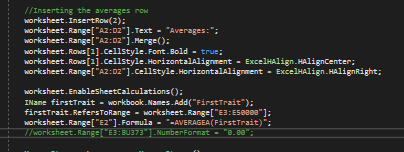We use cookies to give you the best experience on our website. If you continue to browse, then you agree to our privacy policy and cookie policy.# Formulas for Excel

Hello,

I am working on exporting my data from the app to an excel sheet. I have the export working, but now i have to add a row that averages the rows. I have a bunch of columns that i am exporting. The thing I am having trouble with has 2 parts.

1. I was able to create an additional row no problem. I was also able to enter a formula into the cell, but the problem comes when the excel sheet opens. All the "number" data is in the excel sheet as text and because of that the actual formula just returns 0. But once i convert all the "numbers" to an actual number then the formula works great. How do I get the data in the excel sheet to be Numbers and not Text
2. The second part of my issue is that I am currently doing the following to get the one formula to show up:But I have several columns that would need to have the averages calculated. Is there a more efficient way of doing this so I don't need to do this for every single column i have in my excel sheet?
I look forward to your responses.

Thank you,
Dylan

3 Replies

DB Dilli Babu Nandha Gopal Syncfusion Team December 14, 2018 12:25 PM

Hi Dylan,

Query
Formula calculation issue
We are able to reproduce the scenario from the below cases.
1. You may have used IRange.Text property to set cell values while importing data to Excel worksheet. So, the number values are set as text. We suggest you to use the IRange.Numberproperty to set the number values.  This will enable the calculation of cell values in Excel document while opening.
2. When you use the IWorksheet.ImportData(IEnumerable arrObject, int firstRow, int firstColumn,bool includeHeader) method, the object may contain a property of string type which holds a number value. For this, please use the below code. This will change the cell from text type to number type.

Code snippet:
 ExcelEngine excelEngine = new ExcelEngine();   IApplication application = excelEngine.Excel;   IWorkbook workbook = application.Workbooks.Open(GetFullTemplatePath("Create.xlsx"));   IWorksheet worksheet = workbook.Worksheets;      string value = worksheet["A2"].Value;   string value1 = worksheet["A3"].Value;      worksheet["A2"].Value = "";   worksheet["A3"].Value = "";      worksheet["A2"].Value = value;   worksheet["A3"].Value = value1;      workbook.SaveAs("Output.xlsx");

Average calculation for several columns
You can loop through the columns and set the average for all the columns using IWorksheet.Range[int firstRow,int firstCol,int lastRow,int lastColumn] property instead of using the name of the cell every time.
Please refer the code snippet below.

Code snippet:
 ExcelEngine excelEngine = new ExcelEngine();   IApplication application = excelEngine.Excel;   IWorkbook workbook = application.Workbooks.Open();   IWorksheet worksheet = workbook.Worksheets;      int firstCol = 5, lastCol = worksheet.UsedRange.LastColumn;   int noOfCol = lastCol - firstCol;   for (int i = 1; i <= noOfCol; i++)   {       IName name = worksheet.Names.Add("Trait_" + i);       name.RefersToRange = worksheet[3, firstCol, 50000, firstCol];       worksheet[2, firstCol].Formula = "=AVERAGE(" + name.Name + ")";       firstCol++;   }

Kindly try this and let us know whether it helps.

Regards,
Dilli babu

DW Dylan Wegner December 17, 2018 07:30 AM

Hello Dilli,

The Average calculation for serval columns worked great. Thank you.

However I am looking at your suggestion to the Formula calculation issue and it doesn't seem practical for me to go through all the values in the spreadsheet and get the value then set the column to an empty string and then put the value right back in. I have a lot of rows and that just doesn't seem efficient. If i have 1000 rows and I have to write 3 lines of code for each. That's a lot of lines. Can you help me figure out a way that would be more efficient?

Thank you,
Dylan

PK Prakash Kumar D Syncfusion Team December 18, 2018 08:04 AM

Hi Dylan,

The issue can be fixed by following ways.

1.      When you are opening an existing document, replacing the range with empty value and restore the original value using IRange.Value is the only way when the number value is set as string

2.      By changing the property type from string to integer will change the cell value type while importing data from CLR Objects

3.      If the data is imported using data table, then we suggest you to set the preserve types to FALSE in the method IWorksheet.ImportDataTable( DataTable dataTable, bool isFieldNameShown, int firstRow, int firstColumn, bool preserveTypes ). This will import the data based on the data table cell value type. Please refer the code snippet below.

Code snippet:
 ExcelEngine excelEngine = new ExcelEngine();  IApplication application = excelEngine.Excel;  IWorkbook workbook = application.Workbooks.Create(1);  IWorksheet worksheet = workbook.Worksheets;    DataTable reports = new DataTable();  reports.Columns.Add("SalesPerson");  reports.Columns.Add("SaleJanJune");  reports.Columns.Add("SaleJulyDec");    reports.Rows.Add("Andy Bernard", "12400","25500");  reports.Rows.Add("Jim Halpert", "45000", "35000");  reports.Rows.Add("Karen Fillippelli", "34000", "98000");  reports.Rows.Add("Phyllis Lapin", "70000", "90000");  reports.Rows.Add("Stanley Hudson", "80000", "90000");    worksheet.ImportDataTable(reports, true, 1, 1, false);

If it does not resolve your issue, we request you share us the workflow details about how you import the data into worksheet or create the Excel document from your application with us. We will analyze further on this and provide you a solution at earliest.

Regards,
Prakash Kumar